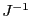Next: Functions Up: Implementation Previous: Implementation   Contents

Return value

• -1: A singular matrix has been found during the scheme (not applicable if we use Newton with a constant)
• 0: Newton has not converged after MaxIter iteration
• 1: Newton has converged toward solution Input
• 2: only valid for the implementation in which the function evaluation return an interval vector. It has not been possible to find a solution such that all the mid point of the interval evaluation of the function was at a distance less than Accuracy from 0. However the procedure will return as solution the point obtained during Newton iteration for which the interval evaluation of all the function include 0 and has the minimal average value for the width of the interval evaluation of the functions.

Jean-Pierre Merlet 2012-12-20